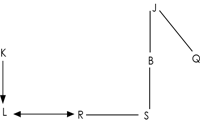# Qualcomm Reasoning Question

DIRECTIONS for question 1 to 5: The question is based on the five three-digit numbers given below:
568     378     654     496     476
1. What will be the resultant if third digit of the highest number is subtracted from the second digit of the smallest number?
1. 3
2. 5
3. 4
4. 2
5. 1
568 378 654 496 476
According to question, highest number of the series is 654. Smallest number
of the series is 378. Third digit of the highest number which is 4, is
subtracted from the second digit of the smallest number which is 7 then result 7-4=3.
2. If in all the numbers the position of first digit is interchanged with the third digit within the number, how many numbers thus formed will be divisible by 5?
1. 2
2. 1
3. 3
4. More than three
5. None of these
If in all the numbers the position of first digit is interchanged with the third
digit within the number, the series is 865 &nbs;p    873 &nbs;p    456 &nbs;p    694 &nbs;p   674 Only 865 is the number
which is divisible by 5.
1. If all the digits in each number are arranged in descending order within the number and the numbers thus formed are arranged in descending order from left to right, which of the following will be the third number from the left end?
1. 568
2. 476
3. 378
4. 496
5. 654
If all the digits in each number are arranged in descending order within the
number and the numbers thus formed are arranged in descending order
from left to right, then series is 964 973 865 764 654
865 is the third number from left. therefore, 568 is answer.
2. If 1 is subtracted from every even digit in each of the numbers, how many numbers thus formed will have a digit appear twice?
1. None
2. More than three
3. One
4. Two
5. Three
If 1 is subtracted from every even digit in each of the numbers, Series is :
557 &nbs;p    377 &nbs;p    553 &nbs;p    395 &nbs;p    375
Thus, there are 3 numbers which have a digit appearing twice (i.e., 557, 377 and 553).
3. If '2' is added to the first digit of each number and '2' is subtracted from the middle digit and third digit of each number, the sum of digits of how many numbers thus formed will be equal to 17?
1. Four
2. Two
3. None
4. One
5. Three
If '2' is added to the first digit of each number and '2' is subtracted from the
middle digit and third digit of each number, then series is,
746 556 832 674 654
7+4+6=17 & 6+7+4=17. There are two numbers whose sum of digits is 17.
4. If only one meaningful English word can be made with the fourth, the sixth and the ninth letters of the word COMPUTERISE, {when counted from left to right) using each letter only once, then which of the following will be the second letter of the word from the right. If no such word can be formed, then your answer is 'X'. If more than one such word can be formed then your answer is 'Z'?
1. X
2. P
3. Z
4. I
5. None
Meaningful word can be made from P, I & T are PIT and TIP. Since, more than one such word can be formed thus correct answer is Z.
5. If '1' is subtracted from each even digit and '2' is subtracted from each odd digit in the number 3724568 then how many digits will appear more than once in the number thus formed?
1. None
2. One
3. Three
4. More than three
5. Two
If '1' is subtracted from each even digit and '2' is subtracted from each odd digit in the number
3724568, Number become 1513357.1,3 and 5 digits appear more than once. Answer is three.
6. In a certain code language, CURED is coded as #6%7*and similarly ROADS is coded as %43*9. How will CODES be coded in the same code language?
1. #4*79
2. #*379
3. #4%63
4. #4*%9
5. *73#6
6. 31 days
C- #
U-6
R-%
E-7
D-*
And also code for
R-%
O-4
A-3
D-*
S-9
CODES are coded in the same code language #4*79.
DIRECTIONS for question 9 to 10:Read the question and answer these questions carefully.

L is the only child of K. R is married to L. S is the sister of R. S is the only daughter of B. J, the father of B has only two children. Q is the daughter of J.

7. How is J related to S?
1. Grandfather
2. Brother-in-law
3. Cousin
4. Father-in-law
5. 32